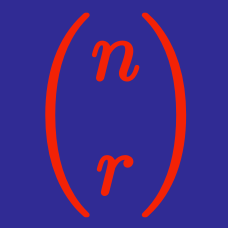Algebra

# Properties of Binomial Coefficients

What positive integer $n$ satisfies ${{n+2} \choose 11}=109 \cdot {n \choose 9}?$

Caroline has $417$ coins, no two of which are the same. The number of ways she can choose $89$ of these coins is the same as the number of ways she can choose $k$ of these coins for some $k > 89.$ What is the value of $k?$

What is the arithmetic mean of the following sereis: ${ 8 \choose 0 }, { 8 \choose 1 }, { 8 \choose 2 }, \cdots, { 8 \choose 8 }?$

What is the value of positive integer $n$ satisfying ${26 \choose n}={26 \choose {n-4}}?$

If ${n+4 \choose 2}+{n+3 \choose 2}=25,$ what is the value of $n?$

×